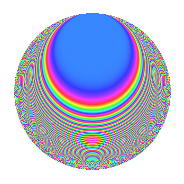# Properties

 Label 108.3.kLevel 108 Weight 3 Character orbit k Rep. character $$\chi_{108}(5,\cdot)$$ Character field $$\Q(\zeta_{18})$$ Dimension 36 Newform subspaces 1 Sturm bound 54 Trace bound 0

# Related objects

## Defining parameters

 Level: $$N$$ $$=$$ $$108 = 2^{2} \cdot 3^{3}$$ Weight: $$k$$ $$=$$ $$3$$ Character orbit: $$[\chi]$$ $$=$$ 108.k (of order $$18$$ and degree $$6$$) Character conductor: $$\operatorname{cond}(\chi)$$ $$=$$ $$27$$ Character field: $$\Q(\zeta_{18})$$ Newform subspaces: $$1$$ Sturm bound: $$54$$ Trace bound: $$0$$

## Dimensions

The following table gives the dimensions of various subspaces of $$M_{3}(108, [\chi])$$.

Total New Old
Modular forms 234 36 198
Cusp forms 198 36 162
Eisenstein series 36 0 36

## Trace form

 $$36q - 9q^{5} + 6q^{9} + O(q^{10})$$ $$36q - 9q^{5} + 6q^{9} + 36q^{11} + 45q^{15} + 42q^{21} - 18q^{23} - 9q^{25} - 18q^{29} + 45q^{31} - 153q^{33} - 243q^{35} - 123q^{39} - 198q^{41} + 90q^{43} - 333q^{45} - 243q^{47} + 72q^{49} - 99q^{51} + 243q^{57} + 252q^{59} - 144q^{61} + 381q^{63} + 747q^{65} + 108q^{67} + 585q^{69} + 324q^{71} - 63q^{73} + 597q^{75} + 495q^{77} + 36q^{79} - 54q^{81} - 27q^{83} - 180q^{85} - 441q^{87} - 567q^{89} + 99q^{91} - 699q^{93} - 1044q^{95} - 216q^{97} - 945q^{99} + O(q^{100})$$

## Decomposition of $$S_{3}^{\mathrm{new}}(108, [\chi])$$ into newform subspaces

Label Dim. $$A$$ Field CM Traces $q$-expansion
$$a_2$$ $$a_3$$ $$a_5$$ $$a_7$$
108.3.k.a $$36$$ $$2.943$$ None $$0$$ $$0$$ $$-9$$ $$0$$

## Decomposition of $$S_{3}^{\mathrm{old}}(108, [\chi])$$ into lower level spaces

$$S_{3}^{\mathrm{old}}(108, [\chi]) \cong$$ $$S_{3}^{\mathrm{new}}(27, [\chi])$$$$^{\oplus 3}$$$$\oplus$$$$S_{3}^{\mathrm{new}}(54, [\chi])$$$$^{\oplus 2}$$

## Hecke characteristic polynomials

There are no characteristic polynomials of Hecke operators in the database